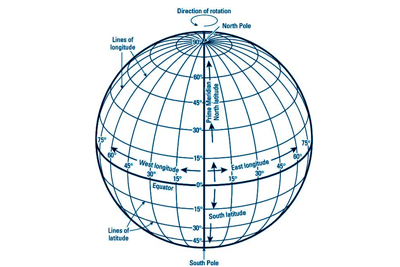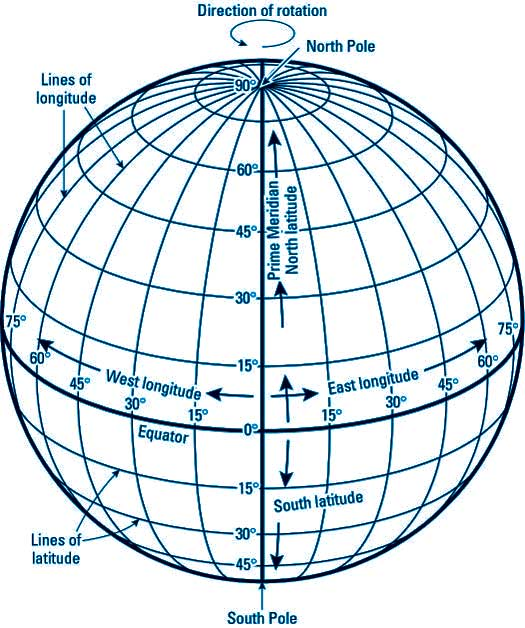The Earth Grid - Physical Geography
11-09-2018    5226 timesThe Earth is shaped like a sphere. So the globe is the best option to represent the Earth most accurately. In order to locate correctly each and every place on the surface of the Earth, a network of horizontal and vertical lines is drawn on the globe. This network of systematic lines is called the ‘Earth Grid’. Since the lines are drawn on a globe, each horizontal line is a circle and each vertical line is a semi circle. The vertical lines are called the ‘Meridians of Longitude’, while the horizontal lines are ‘Parallels of Latitude’. The point, where the lines intersect, pinpoints the location of a place.

We are aware that the rotating Earth is spinning round its axis. The axis or the imaginary narrow rod, on which the globe turns, pierces the globe at two fixed points, the ‘North Pole’ and the ‘South Pole’. The exact mid way between the two poles is the ‘Equator’, which is the imaginary line (actually a circle) running round the middle part of the Earth. The Equator divides the Earth into two halves, called the ‘Northern Hemisphere’ and the ‘Southern Hemisphere’. The word ‘Hemisphere’ means half of a sphere or a globe.